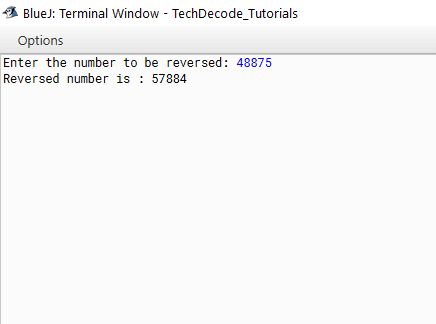# How to Reverse a Number in Java using for loop

If you have a foundation in computer science, broadening your technical knowledge is the finest thing you can do. However, your programming abilities are the most important, and you must continue to polish them in order to become an effective programmer in the future. Though there are several things to focus on, one area in particular that you must emphasise is the world of data structures. Data structures, in general, are unique techniques to solving issues that use the least amount of computer resources. There are a variety of data structures that you can study and use in general. To keep things easy, we’ll start with some basic programmes that you’ll need to master before moving on to more advanced algorithms. So today let us start with How to Reverse a Number in Java using for loop.

## What’s The Approach?

• Import the java.util package into the class.

• Now within the main method create a new object of Scanner class. for example  `Scanner sc=new Scanner(System.in);`

• Use the Scanner class to get the input from the user.

• Now create a for loop with no initial condition.  Which will execute till the value of the number entered is equal to zero . The number changes to number/10 after iteration.

• Inside the For loop have a variable that will extract the last digit of the number using the modulus (%) operator.

• Store that extracted digit to the reversed number variable, multiply it by 10 after every iteration and add the newly extracted last digit

• Print the Reversed number.

Also Read:Print Prime Factors of a Number in Java

### Java Program to Reverse a Number Using For Loop

```/*
* TechDecode Tutorials
*
* How to Reverse a number using for loop in Java
*
*/

import java.util.*;
public class Reverse_ForLoop
{
public static void main(String args[])
{
// Creating an object of Scanner class
Scanner sc=new Scanner(System.in);
System.out.print("Enter the number to be reversed: ");
// taking imput from  the user
int num=sc.nextInt();
// integer to store reversed number
int rev=0;

//Note:- We are keeping the initialization part of for loop empty

for( ;num != 0; num=num/10)
{
// extracts the last digit of the original number
int last_digit = num % 10;
// stores and updates the reversed number after every iteration
rev = rev * 10 + last_digit;
}
// printing of reversed number
System.out.println("Reversed number is : " + rev);
}
}
```

`Input:    ``48875`

`Output:   ``57884`